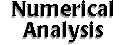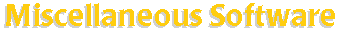[an error occurred while processing this directive] Miscellaneous software that help perform the numerical analysis. Be sure to look at Source Code Repositories subsection first when searching for free software. Search SAL: Miscellaneous Software   (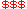Commercial,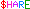Shareware,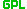GPL) General/Basic Numerical Libraries: aimLib-- a C++ mathematics library. C++ Classes List -- several C++ classes for numerical analysis. CCMATH-- a mathematics library coded in the C-language. Cephes -- C language library for special functions of mathematical physics. CERNLIB -- collection of general purpose programs for mathematical or data-handling problems. C/Math Toolchest-- C/C++ library for scientific and engineering applications. Chorale ToolBox-- numerical toolbox for Matlab/Octave. CMLIB -- a collection of Fortran subroutine for mathematics and statistics. Colt -- open source libraries for high performance scientific computing in Java. CPML -- a replacement libm library for Alpha Linux with better performance. GNUMATH(gnussl)-- a numerical mathematics library for scientific applications. GSL (GNU Scientific Libaray)-- a collection of numerical routines for scientific computing. Harwell Subroutine Library-- a suite of Fortran subroutines for scientific computation. IMSL Math/Stat Libraries-- more than 900 FORTRAN numerical subroutines. IMSL C Numerical Libraries-- math/statistics numerical subroutines for C programming. jCrunch-- Java class libraries for robust and fast mathematical capabilities. JNL -- a numerical library for Java. LHC++-- OO software library to replace CERNLIB. libffm -- fast and free math routines for Linux/AXP.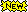Matpack-- a C++ numerics and graphics library. Multipack-- math Fortran routines linked to Python. NAG C Libraries-- a large collection of algorithmic routines written in C. NAG Fortran 77 Libraries-- Fortran 77 routines for mathematical and statistical computation. NAG FL90plus and fl90 Fortran 90 Libraries-- Fortran 90 libraries. PORT -- a collection of Fortran 77 routines for many traditional areas of mathematical software. Scientific Math Library in C++ -- extensive collection of classes and routines. Schelab -- a numerical analysis library for Scheme. Scientific Python modules -- Python modules suitable for scientific research. sl++-- a C++ object oriented numerical library. SLATEC -- common mathematical library. SLICOT -- a general purpose basic control library. TCBI NumLib-- templated library of numerical base calsses for basic data structures. WNLIB -- a C subroutine library that contains numerous tools useful for programming pratice. XSC -- language extensions for scientific computation for modern numerical software. C-XSC++-- a C++ class library for extended scientific computing. Linear Algebra: BLAS -- high quality building block routines for performing basic vector and matrix operations. ATLAS -- a self-optimizing BLAS implementation. C_BLAS 123-- Pentium optimized BLAS implimentation for C. DGEMM for Alpha Chip-- highly optimized dgemm implementation for Alpha/Linux. LAPACK and BLAS libraries for Linux -- ELF shared libraries of linear algebra routines. Pentium Optimized BLAS-- a project of optimized BLAS implementation. PHiPAC -- BLAS-compatible matrix-matrix multiply generator using ANSI C. Optimized Libraries for Pentium Pro -- BLAS, FFT, Extended Precision, etc. Blitz++ -- a C++ template class library for scientific computing. BPKIT -- a toolkit of block preconditioners for the iterative solution of linear systems. C++ Matrix Class -- a simple, fast, efficient C++ Matrix class designed for scientists and engineers. C++ Tensor Class -- a recursive class template definition for N-dimensional arrays. C_EisPack-- full implementation of EISPACK optimized for C/C++. CAM C++ Class Libraries -- graphics, matrix/vector and symbolic function classes. Cheap Matrix-- a C++ Matrix class optimized for memory. Expokit -- software for computing small dense and large sparse matrix exponentials. GISOLV-- general iterative solver for large scale linear system. GMRES/FGMRES -- implementation of GMRES iterative linear solvers. IML++-- C++ templated library of iterative methods for solving linear systems. ISIS++ -- iterative scalable implicit solver in C++. JAMA -- a basic linear algebra package for Java. JAMPACK -- a class library for manipulating matrices in Java. Iterative Linear Solvers for Finite Volume/Finite Difference Equations LAPACK -- solving linear (includes least-square) equations, eigenvalue and singular value problems. LAPACK-D-- dynamically loadable version of LAPACK library. LAPACK and BLAS libraries for Linux -- ELF shared libraries of linear algebra routines. LASPack -- solving large sparse systems of linear equations like those from solving PDE.LinAl-- a simple, easy to use and efficient C++ linear algebra library. LinAlg -- basic linear algebra and optimization C++ classlib.matgen-- a command line matrix generator. Matrona -- a small C++ class library with widely-used algorithms of matrix algebra. MatView -- a scalable sparse matrix viewer. Meschach-- a C-language library of routines for performing matrix computations.MET-- a C++ matrix class library promoting the notational convenience of linear algebraic. MTL -- a high-performance generic component C++ library for matrix. MV++ -- a small/efficient set of concrete vector and simple matrix C++ classes. Newmat -- a C++ matrix library. MultiScale Libaray-- routines for linear algebra, transformation and wavelet. SparseLib++ -- a C++ class library for efficient sparse matrix computations. SVMT-- the C++ scalar, vector, matrix and tensor class library. Template Numerical Toolkit (TNT) -- a numeric library for scientific computing in C++ (mainly linear algebra routines). Differentiation/Integration: ADIFOR -- automatic differentiation of Fortran codes. BVP Software -- solving boundary value problem for ODE. Cubpack++ -- a C++ package for automatic two-dimensional cubature. DNA -- fast numerical solution of ODE in Maple. FADBAD/TADIFF -- C++ package for automatic differentiation and Taylor expansion. GELDA -- general linear differential algebraic equation solver. Godess -- a generic ordinary differential equations solving system. Integration Applet -- graphs user-supplied function and computes the area under the curve.IVP Test Set -- Fortran 77 test set for initial value problems. Numerical Integration -- collection of FORTRAN code for numerical computation of integrals. Ode-- numerical solution of ordinary differential equations. ODE++ -- a C++ class library for ordinary differential equations.ODE Fortran Codes -- Fortran 77 programs for various ODE problems. PCOMP -- automatic differentiation. PseudoPack -- numerical differentiation by pseudospectral methods. Scarab-- numerical integrator for ODE. TAMC -- generate Fortran source for 1st order derivatives out of a Fortran routines. TIDE -- a collection of C++ classes for differential equations. XTC -- a tool for modeling spatial evolution equations (PDE, integro-differential equations). Transform: Optimized Libraries for Pentium Pro -- BLAS, FFT, Extended Precision, etc. benchFFT -- a program to compare FFT implementations. djbfft -- a fast code for small power-of-2 complex DFTs on a Pentium. FFTW-- a C subroutine library for performing the Discrete Fourier Transform. FXT -- C++ library for various transformation. LiftPack -- a C library for the fast lifted wavelet transform (FLWT). MultiScale Libaray-- routines for linear algebra, transformation and wavelet. Radix-2 FFT -- radix-2 DFT of a sequence, or Fourier integral of an evenly tabulated function. SPLIB -- spectral method routines. Uvi_Wave -- a set of Wavelet Processing based functions implemented under Matlab and khoros 2. WAILI-- a wavelet transform library which includes some basic image processing operations. WavBox-- wavelet transforms and adaptive wavelet packet decompositions. WaveLab -- library of MATLAB for wavelet analysis. wvlt -- a small set of routines that allow the user to manipulate wavelets. Arithmetics/Multi-Precision: apfloat -- C++ arbitrary precision arithmetic package. doubledouble-- implements approximately 30 decimal place floating point arithmetic. FreeLIP -- a large integer package to factor the 129-digit number RSA-129. GMP (GNU MP)-- a library for arbitrary precision arithmetic. hfloat-- library for floating point numbers of extreme precision. JUMP -- Java class library for unlimited precision computations. LInteger -- a set of C++ libraries supporting multiple precision integers. Mpexpr -- brings multiple precision math for Tcl. MPFUN -- Fortran code for arithmetic computations to arbitrary numeric precision. MPFUN++ -- a C++ implementation of MPFUN for calculation in arbitrary numeric precision. Piologie -- library for arbitrary precision arithmetic for natural/integer/rational numbers. SoftFloat -- implementation of IEC/IEEE Standard for Binary Floating-point Arithmetic.XR -- an implementation of exact real arithematics. Interpolation/Approximation: C++ NURBS Library-- a C++ library for non-uniform rational B-splines curves and surfaces. DT_NURBS -- a non-unofirm rational B-spline geometry subroutine library. GUL -- geometry utility library for interpolation and approximation with NURBS surfaces. kspline -- a library which provides spline functions. NURBS Library -- Non-uniform rational B-splines library. NURBS++-- a C++ library to manipulate and create NURBS curves and surfaces.NURBS Toolbox-- routines for the creation, and manipulation of NURBS. SCRIP -- a spherical coordinate remapping and interpolation package. Siscat-- an object-oriented numerical library for approximation/interpolation of scattered data. Snurbs-- simple NURBS libaray and a viewer. Spherekit -- toolkit for spatial interpolation/comparison of spatial interpolation algorithms. spline -- program for fitting a set of points using splines under tension. Random Number Generator: A Gaussian Random Number Generator -- with fast time-correlated feature. ENT -- a pseudorandom number sequence test program. Mersenne Twister -- a fast pseudorandom number generator. Newran -- a C++ random number library. pLab -- an object oriented system for generating and testing random numbers. PRNG -- a C library for the generation of pseudorandom numbers. RngPack-- a pseudorandom number generator package for Java. RNPL -- rapid numerical prototyping language help scientists solve equations quickly. Others: ALFPACK -- Fortran subroutines for computing associated Legendre functions. C++SIM -- an object-oriented simulation package written in C++. Chaos Classes-- C++ classes for use in the study of chaotic dynamical systems. CLAWPACK -- conservation laws software package. CONTENT -- dynamical system software with differential equation solvers and tools. cspX-- time series analysis algorithms for analyzing chaotic or regular data sets. DsTool -- computational environment for dynamical systems. Enclosing Ball Software -- calculates the smallest enclosing ball of a set of points or a set of balls. Evolvuton and Other Software -- C++ software components for evolutionary computation and others. FINDER-- pricing financial derivatives using low discrepancy methods. GAIO -- a software package for the numerical analysis of dynamical systems. IAsolver-- the Brandes interval arithmetic constraint solver implemented in Java. LASSPTools -- numerical and graphical enhancements to Unix. A Matlab Differentiation Matrix Suite -- solving differential equations by the spectral collocation method. MCSim-- a package for Monte Carlo stochastic simulations. MODFIT -- parameter estimation in Laplace transforms, ordinary/algebraic differential equations. NAO -- objects for easy creation of numercial analysis software. NumExp-- GTK+ based mathematical calculation program. OAX -- a spacial objective analysis program. Orderpack -- Fortran 90 code for general and specialized ordering problems. PETE -- portable expression template engine.The Polynomial Toolbox-- a MATLAB toolbox for polynomials, polynomial matrices and their applications. PROFIL/BIAS -- a C++ interval arithmetic class library. PDEFIT -- parameter estimation in diffusion equations. SHDOM -- spherical harmonic discrete ordinate method for atmospheric radiative transfer. SLEIGN -- compute eigenvalues, eigenfunctions of regular/singular Sturm-Liouville (S-L) problems. SYSFIT -- parameter estimation in systems of nonlinear dynamical equations. TENSPACK -- nonlinear equation solving with tensor methods. TONS -- to produce an environment for numerical calculations. TRANSIENT-- solving arbitrary, nonlinear, parabolic PDEs in space and time. UniCalc -- a universal solver for arbitrary algebraic systems of equations and inequlities. XPP-Aut -- solving various equations arised in dynamical systems. Also Check Out: Algae-- a high-level interpreted language for numerical analysis. C-Mix -- a program specializer for making faster C code. CGAL -- a C++ software library of geometric data structures and algorithms. CMAT -- an exact arithmetic matrix calculator program. Emu/fdtd -- a 3D Maxwell's equation solver. EiffelMath from ISE Eiffel-- object-oriented library for numerical and scientific computation. ixlib-- C++ library with regular expressions, automatic arrays, garbage collection, matrix handling, planar geometry.JavaView -- a 3D geometry viewer and numerical software library Java. LCS -- Monte Carlo codes for simulating particles in an energy regime from TeV to thermal energies. Matrix-- a Matlab compatible C++ matrix class library. SigLib DSP Library-- DSP, Signal and image processing software and libraries. SILOON -- scripting interface languages for object-oriented numerics.Optimization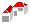SAL Home   |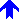Numerical Analysis